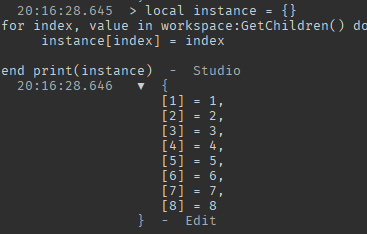# Get instance from a pairs or ipairs

Im trying to put in a variable a instance that I got from using:

``````local instance = {}
for index, value in workspace:GetChildren() do
instance[index] = value

end
``````

I wanna get the instance instead of the value, for example it’s giving me “Baseplate”.

1 Like

you can just do

``````instance[index] = index
``````

or

``````instance[value] = value
``````

depending on which you mean

1 Like

it will give me this:can you further explain what you’re trying to do? Let me know if this is correct

``````local instance = {}
for index, value in pairs(workspace:GetChildren()) do
table.insert(instance, value)
end
``````

What do you mean instead of the value you want the instance when the instance is the value?

1 Like

well after im trying to use :FindFirstChild()

That should be correct. What problems are you getting from that code?

well that’s more complicated, I use a module to stock the loop’s data, in there I detect when a player die and if he respawn we will teleport him in a special place, it’s a custom spawnpoint basically. Here is the module. The module’s parent is my script. The LastChanged option is changed when the player touch another checkpoint.

``````local players = game:GetService("Players")
local class = {}

local userId = player.UserId

class[userId] = {}
class[userId].LastPlayed = nil

if class[userId].LastPlayed ~= nil then
print(class[userId].LastPlayed)
character:WaitForChild("HumanoidRootPart").Position = class[userId].LastPlayed:FindFirstChild("Attachment").Position -- bug there

end
end)
end)

players.PlayerRemoving:Connect(function(player)
table.remove(class, player.UserId)

end)

return class
``````

Can you actually explain what the problem is, because from the looks of it your code is doing exactly what you want…

This is wrong. `table.remove` is for arrays, and it goes hand-in-hand with `table.insert`. What you are using is a hashmap instead, aka a dictonary. To remove it, you just assign the value to `nil`.

``````players.PlayerRemoving:Connect(function(player)
class[player.UserId] = nil
end)
``````
1 Like

thanks, the problem is that I cannot use :FindFirstChild() after using a value the error is “attempt to index nil with :FindFirstChild”, that logic but I cannot find what the problem is

`.LastPlayed` was set to nil. You never gave it a value.

Then it should not print the error because I have a condition that detect if it’s not nil, so I use it in another script which is the parent of the module.

``````local Checkpoint = require(script.Checkpoint)
``````

at a certain time I use

1 Like